Industrial Data Science
in C# and .NET:
Simple. Fast. Reliable.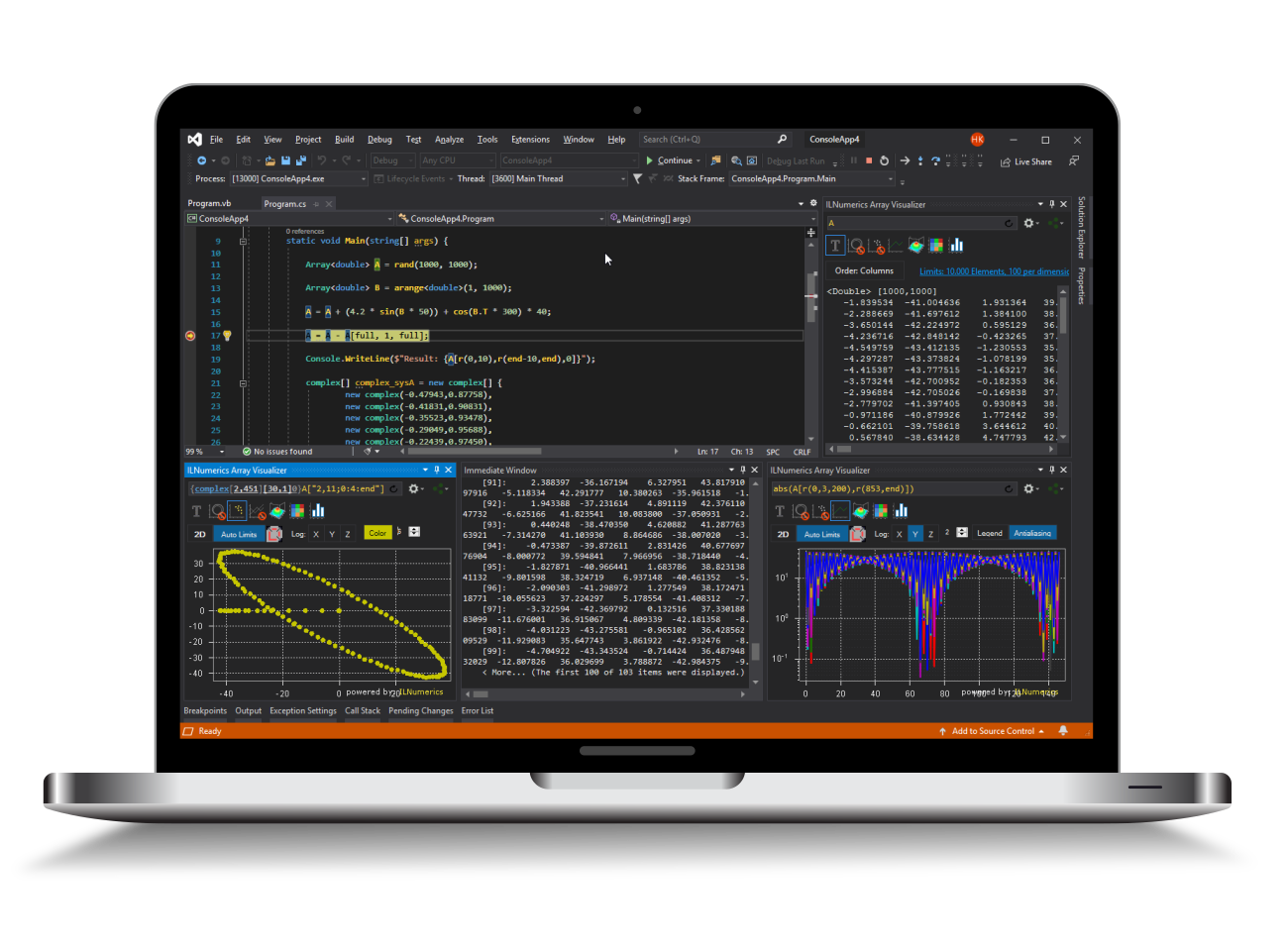ILNumerics - Technical Computing

Modern High Performance Tools for Technical

Computing and Visualization in Industry and Science

# Live Debug Data Plotting for C/C++

ILNumerics Array Visualizer is an interactive, graphical debug tool for Visual Studio. During your debug session it connects to the Visual Studio debug engine and allows immediate insight into the data of your program. Arbitrary array data are plotted in various, interactive chart styles: as 1D and 2D line plots, scatter plots, bar plots, as 3D surface plots, and images. Easily describe the data via arbitrary expressions. Export data in a large number of external formats, including charts, HDF5 and Microsoft Excel. Follow your data with each debug step to quickly find bugs in the algorithm.

The Array Visualizer works for Visual Studio debug sessions in C/C++, FORTRAN, C#, F#, and Visual Basic. This manual was specificly designed for C/C++ users. Consult the language specific manuals for other languages or visit the general Array Visualizer documentation for more details.

## Visualizing Debug Data - 3 Simple Steps

1. When halted in your Visual Studio debug session go to the Visual Studio View menu:

VIEW -> Other Windows -> ILNumerics Array Visualizer

2. Enter the name of an array variable from your current debug scope into the expression text area of the Array Visualizer.

3. (Optional) Choose a plotting type from the list of available 2D and 3D plots.

4. (Optional) Apply further configurations to the plot.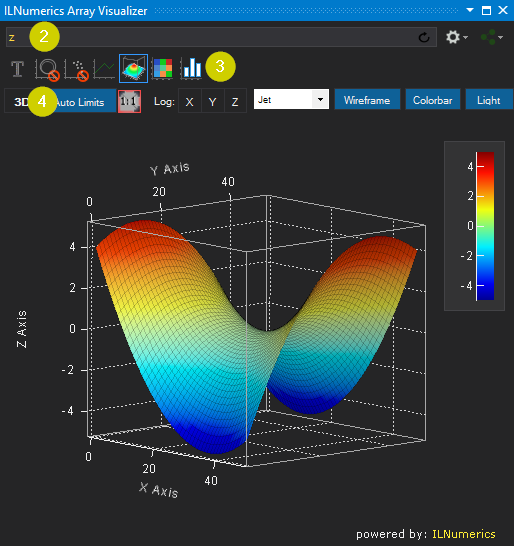## Plotting Array Expressions in C/C++

Let's start by creating a very simple C program:

The content of the variable A at the current statement is plotted by entering A into the Array Visualizer and pressing [Enter]: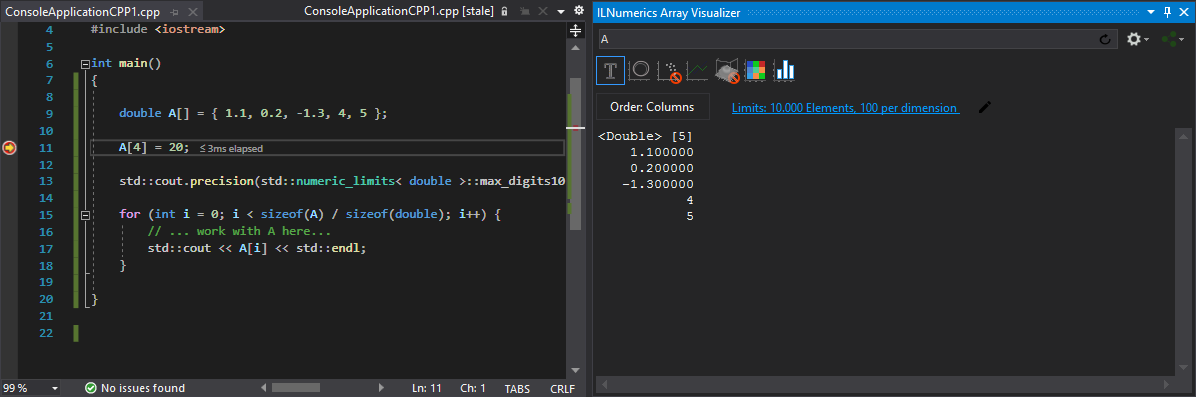Here, the content is shown as text. Click on the line plot tab on the top of the Array Visualizer tool window to show the data as line plot. Note how the plot is linked to the variable: pressing F10 modifies A in the next statement. The change is immediately reflected by the plot. Here, how the window looks after setting line properties: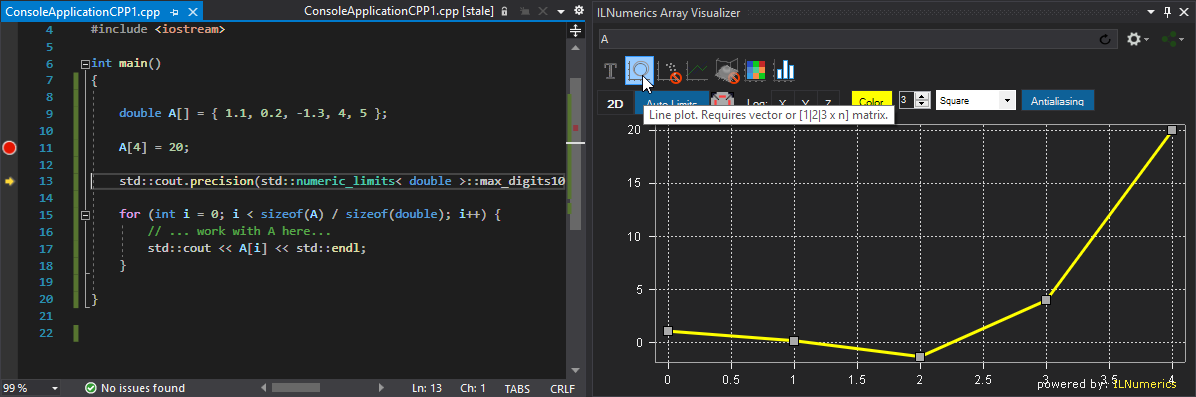### Getting Interactive

No matter, how you interact with the data in your debug session – changes will show up immediately in the array visualizer. One might set the 3rd element of A to the value 10 in the immediate window. See how the plot follows: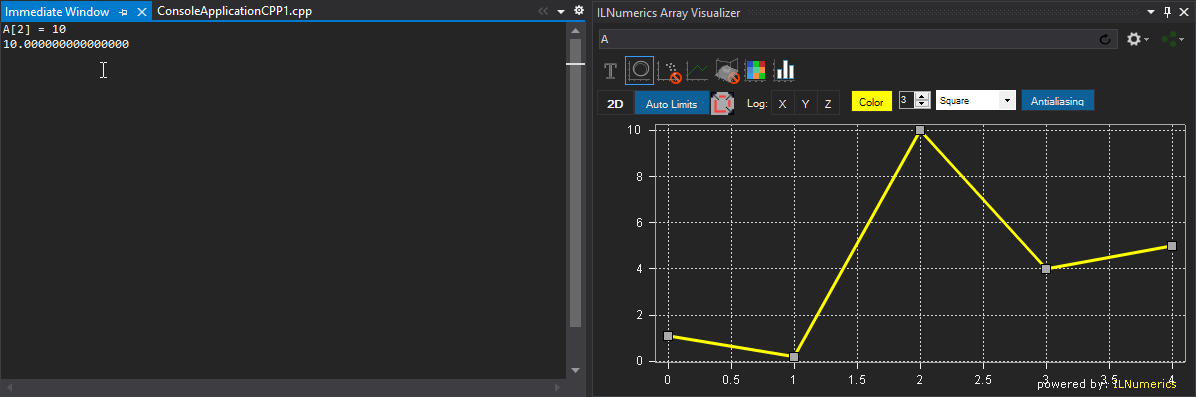### Supported Array Expressions in C/C++

In C/C++ the LNumerics Array Visualizer plots arbitrary expressions yielding the following array types:

• Named arrays: int a[] = {1, 2, 3}, double b = ..., char c, ...
• Array data structurs from std:: namespace: std::vector<T>, std::array<T,n>, std::valarray<T>
• Pointers of any numeric type (int*, float*, ..)
• void* pointers: (basically everything..., including std::complex c[] = ... )
• Arrays of structs of primitve numeric types ('records', user defined types, new in 4.11!)

All primitve numeric element types are supported:

 Floating point data types double float Integral data types unsigned long long unsigned int int unsinged short short char unsigned char, signed char unsigned long long long long __uint64

Expression entered in the Array Visualizer are evaluated in the context of the current debug stack frame. Your program must be halted in debug mode. Any valid C/C++ code can be used as expression for the Array Visualizer, including variables, function calls etc. Basically, everything what would be accepted for evaluating in the Immediate Window is likely to work in the Array Visualizer also.

### N-D Array Plotting Example

Handling matrices and arrays with more dimensions is done similarly: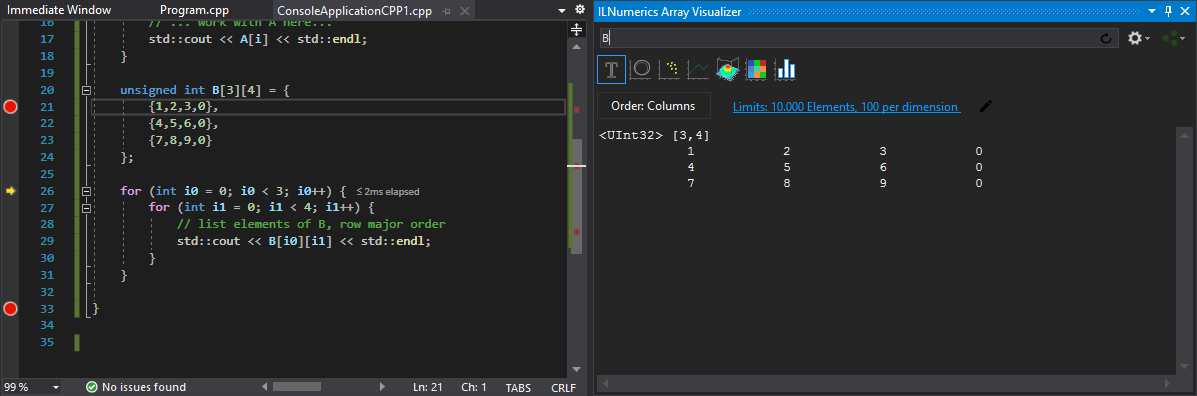The Array Visualizer retrieves the array type and dimension properties from the array. Automatically, if possible.

## Plot Types

Next to the text output, the 2D matrix B from the last example can be visualized with the following chart types:

 XY-Line Plot Scatter Plot Rows Plot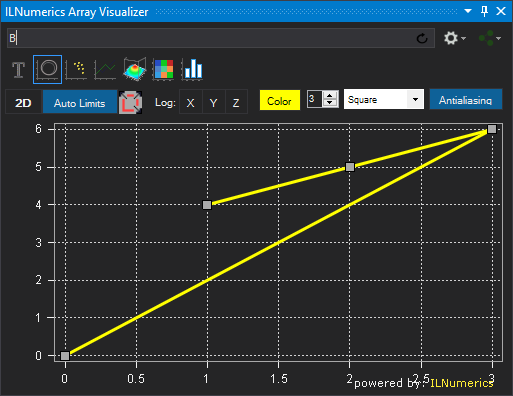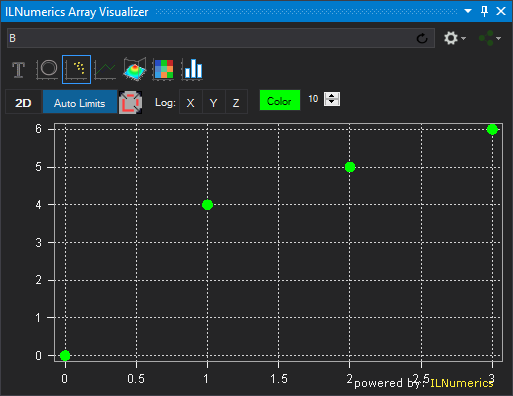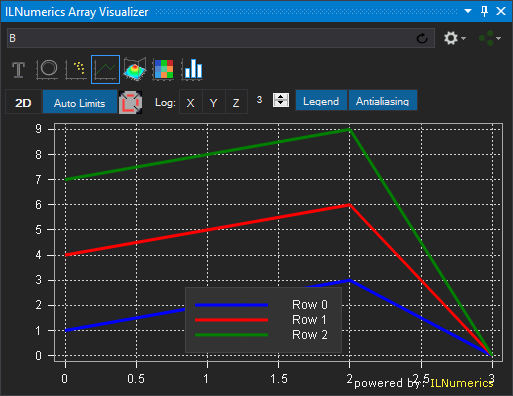Surface Plot ImageSC Plot Bar Plot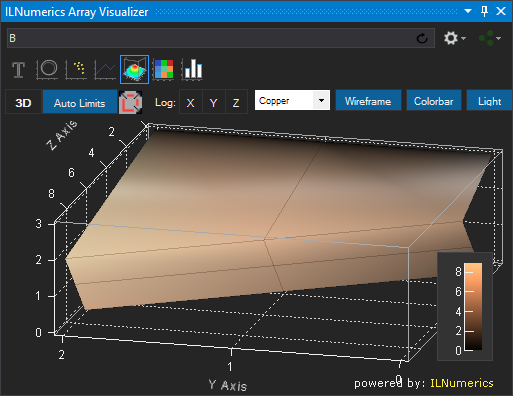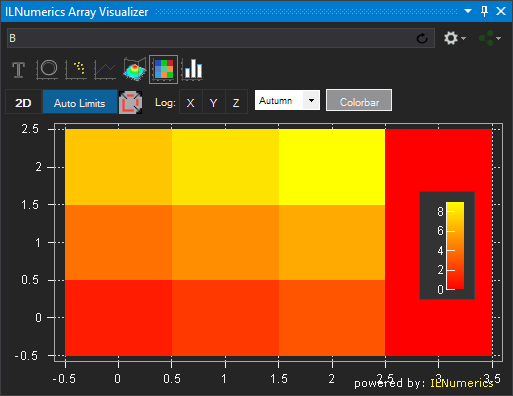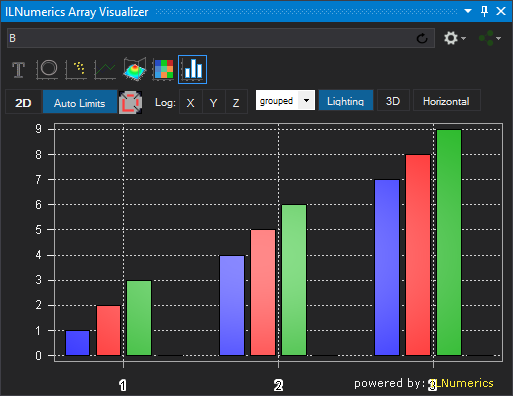The row of iconized buttons near the top of the the Array Visualizer tool window represents individual plot types. Each plot provides a different view of the data. Read more

## Describing C/C++ Memory with Array Descriptors

In the previous paragraphs of this manual, the data to plot were represented as full dense array variables. But many technical applications implement more complex data. In most situations, the interesting data are stored within recordsets, or structs of more complex data types.

Example: potential data type definition for storing 3D measurement data.

Here, 5 data elements of type double are stored for each measurement: the X,Y, and Z coordinates as the position of the measurement and two arbitrary data values. Data1 and Data2 might be used to store the actual value measured or some error range / confidence information computed for the measurement or any other useful value.

In real world applications data structures like this are very popular. Working with them can be cumbersome, especially if the number of measurements grows high. Often enough, developers end up wasting time in printing large lists of numbers into text files or by inspecting individual fields of every single measurement, utilizing data tips in Visual Studio: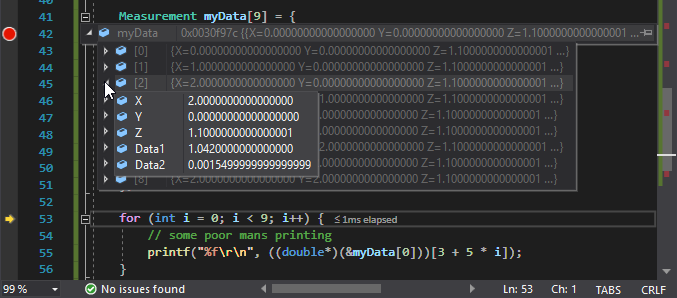The ILNumerics Array Visualizer simplifies things significantly. All we need to do is to provide some 'meta data' about our Measurement[] array. Recall the memory layout of the measurement data, starting at address 0x02DE28A4: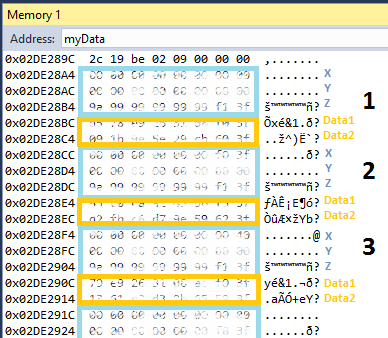We let the Array Visualizer consider the data as double* and enter the following expression:

`(double*)&myData `

I.e.: we acquire a double* pointer for the first element (myData.X). When pressing Enter the Array Visualizer kicks in and asks for more details: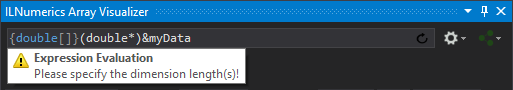Additional details in Array Visualizer are provided by means of array descriptors. One simple way of looking at the data is to consider them as a 9 x 5 double matrix. This can be achieved by providing the dimension lengths '9,5' to the array descriptor and pressing enter.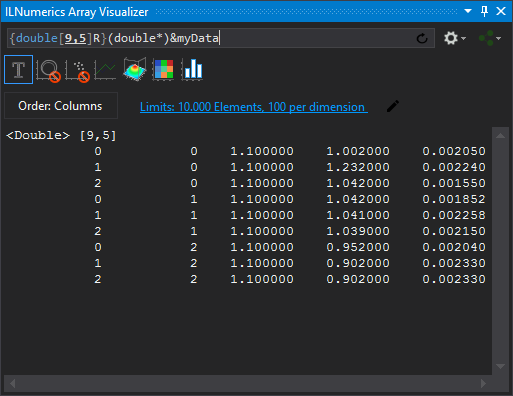More array descriptor trickery allow us to pick individual elements from the struct array and have them plotted in various ways: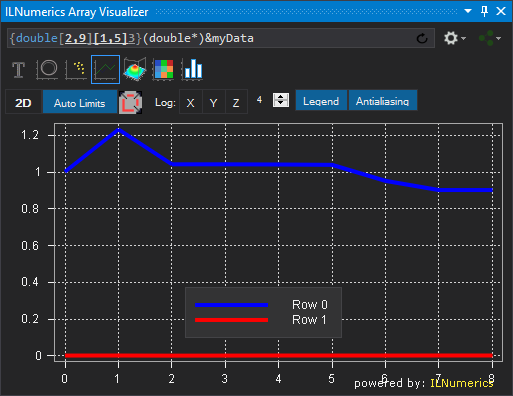Using the array descriptor expression:

`{double[2,9][1,5]3}(double*)&myData`

we extract the Data1 and Data2 members from the struct array and plot their values together as row plots. The array descriptor collects the two 'array' line data for Data1 and Data2 into a matrix with the following properties:

• 2 rows of 9 elements each, row 1: Data1; row 2: Data2.
• Element type is double.
• Data1 and Data2 elements are located by help of the striding information: element spacing between Data1 and Data2 members are 1 for the same measurement. Individual mesurements have an element spacing of 5 double elements.
• Data extraction starts at the first Data1 element (offset: 3).

Let's create another example for our measurement data by visualizing the positions (XY coords) of the measurements. Scatter plots are useful to visualize individual data points, when the data coords come from a matrix with up to 3 rows and each column of the matrix corresponds to one data point.

###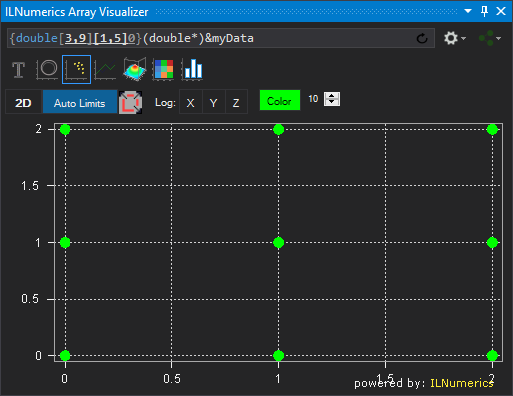This makes the 3D positions of the measurements clearly visible. We show the X-Y positions only here, since we look straight onto the X-Y plane. If there were any useful Z values we would visualize them by switching to the 3D view and rotating the plot with the mouse. Modify the original data and try this yourself!

Another useful view is given by the bar plot tab. It shows the X, Y and Z coordinates as separated groups: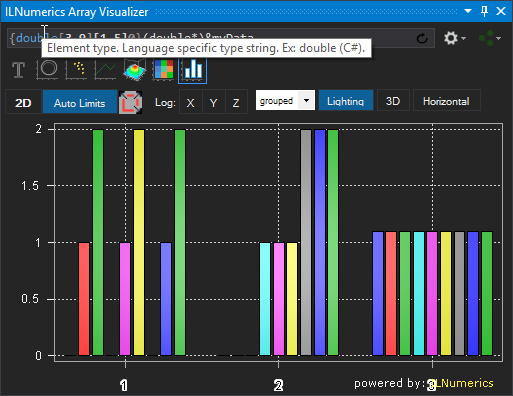Now we could easily add both, Data1 and Data2 as new rows to the matrix - this is left to the reader as exercise.

### Plotting arrays of complex numbers in C/C++

The ability to visualize the content of arbitrary memory segments is a very useful tool. Given a pointer to the data it lets us pick the interesting parts from your debug structures and visualize them in an intuitive way.

One more example of this convenience is given by arrays of complex numbers.

While the Array Visualizer currently is not able to directly accept complex numbers as elements of named arrays, we can again make use of the knowledge how complex data are stored in memory. All popular implementations store the data as double values, interleaved in memory: real1 + imag1 + real2 + imag2 + real3 .... a.s.o. So, in order to plot arrays of complex data, we only need to acquire a pointer to the data array and make use of the array descriptor syntax to describe element type, array length(s) and strides:

`{double[5,2][2,1]}(double*)&C`

This extracts the complex numbers into two columns for real and imaginary parts: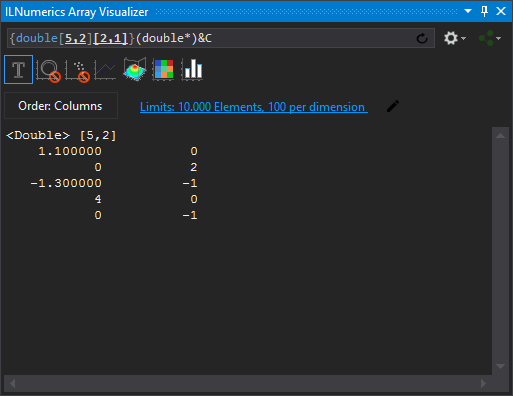Once the numbers are nicely ordered into a suitable array the Array Visualizer is able to visualize the values by means of graphical plots. For example, if we arrange the real and imaginary parts as rows, the row plot type gives: